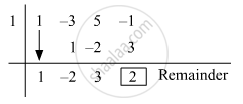Advertisement Remove all ads

# Divide Each of the Following Polynomial by Synthetic Division Method and Also by Linear Division Method. Write the Quotient and the Remainder. - Algebra

Sum
Divide each of the following polynomial by synthetic division method and also by linear division method. Write the quotient and the remainder.
(y^3 - 3y^2 + 5y - 1) ÷ (y - 1)
Advertisement Remove all ads

#### Solution

Synthetic Division:

Dividend = y^3 - 3y^2 + 5y - 1

Divisor = y - 1

Opposite of −1 = 1The coefficient form of the quotient is (1, −2, 3).
∴ Quotient = y− 2y + 3 and Remainder = 2

Linear Method:

y^3 - 3y^2 + 5y - 1

= y^2 (y - 1) + y^2 - 3y^2 + 5y - 1

= y^2 (y - 1) - 2y (y - 1) -2y +5y - 1

= y^2 (y - 1) - 2y (y - 1) + 3(y -1) + 3(y - 1) +3 - 1

= (y - 1) xx (y^2 - 2y + 3) + 2

Concept: Synthetic Division
Is there an error in this question or solution?
Advertisement Remove all ads

#### APPEARS IN

Balbharati Mathematics 1 Algebra 9th Standard Maharashtra State Board
Chapter 3 Polynomials
Practice Set 3.3 | Q 1.6 | Page 46
Advertisement Remove all ads
Advertisement Remove all ads
Share
Notifications

View all notifications

Forgot password?